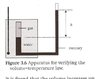# Boyle's Marionette Law:

• ProPM

#### ProPM

Hi,

I am a bit confused over some things:

Is it wrong to say: P = 1 / V? This would imply that P * V would always equal 1 while it can equal a wide range of constants, correct? Therefore, it is better to write P = k / V?

Furthermore, why when we plot P = 1 / V we get a straight line graph? Why do we graph this relation?

1.Correct P=k/V
2.Do you know the general equation for a straight line graph(y=mx+c)?
P is equivelent to y,
1/V is equivelent to x
m is equivelent to k
c=0
One advantage of manipulating an equation so as to plot a straight line graph is that it is easier to draw the line of best fit.

It's called Boyle - Mariotte's Law.

This is a marionette:
[PLAIN]http://marionette.cz/images/T/kasper_osn.jpg[/CENTER] [Broken]

:rofl:​

Last edited by a moderator:
Hi guys, thanks for the answers - Dadface for the objective response and Dickfore for the humorCould you guys help me with two last things if possible?

Ok, firstly, how do we write P * V = k in the y = mx + c form, I mean, the mathematical steps.

And, finally, this is the experiment my book described for the Volume - Temperature Law: Gas is surrounded by water that is heated from below. As it expands, the pressure is kept constant by adjusting the amount of mercury in the tube so that h stays the same. The constant gas pressure is thus the sum of the atmospheric pressure plus the amount pgh.

This is what I understand: The water heats up and therefore, causes the gas to heat up too. As a consequence, the gas will expand but, in doing so, it's pressure would decrease but it is kept constant (how, I don't understand... That and the fact the constant pressure would be the pressure in the mercury plus the atmospheric pressure...)

#### Attachments

•Scan 1.jpg
44.7 KB · Views: 345
$$P V = k$$

You can make it linear in many ways. Personally, I would prefer plotting it on a log-log plot:

$$\log P + \log V = \log k$$

then, take $x = \log V$ and $y = \log P$ and you will get a linear relation. What are the coefficients m and c in this case?

However, you could do it as was suggested in your op, namely solving for P and seeing in what function of V is it proportional.

Ok, got that part, but I am still a bit confused with the experiment.In answer to your first question and in addition to what Dickfore wrote you have already carried out the mathematical steps when you wrote P=k/V.Compare this to y=mx+c.

As for your second question when the gas is heated its pressure tends to rise but the pressure is deliberately kept constant by the method you described.In order for you to understand this fully I suggest you do a bit of reading up on "manometers.

"is kept constant by adjusting the amount of mercury in the tube so that h stays the same. The constant gas pressure is thus the sum of the atmospheric pressure plus the amount pgh." I don't get the whole of this part...

When I looked at the experiment I thought the pressure remained constant because the gas was allowed to expand (for it's pressure was greater than the atmospheric pressure on the other side of the mercury) and it managed to do so until the pressure outside was equal to the pressure of the gas. In the process, the mercury would be moved up and down but I don't get how the book says it involves adjusting the amount of mercury so that h stays the same!

You have been sort of answering your own questions.There is an often used variation of this experiment where P is kept constant at atmospheric pressure by trapping the gas in a capillary by a small liquid and therefore low friction piston(usually sulphuric acid so as to dry the air).With this variation the liquid piston moves until the pressure equalises and becomes(approximately) equal to atmospheric pressure.
Your experiment is more sophisticated and enables you to test constant pressures other than just atmospheric pressure.As I said previously you need to understand manometers(and hydrostatic pressure).Try googling "hyperphysics" then go to manometers.